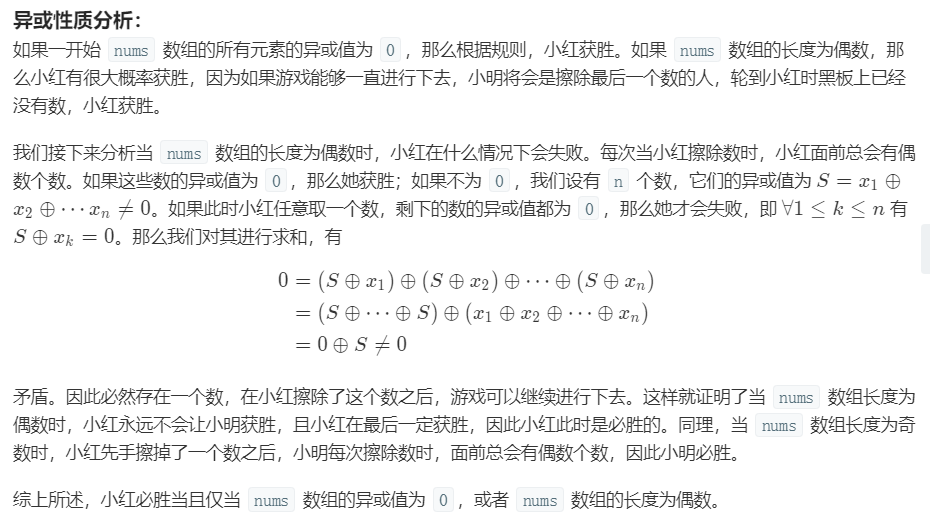# JAVA程序设计：黑板异或游戏（LeetCode：810）

1 <= N <= 1000
0 <= nums[i] <= 2^16

class Solution {
public boolean xorGame(int[] nums) {

boolean[] flag=new boolean[nums.length];

int id=0,num=0;

while(num<nums.length) {

int res=0;
boolean mark=false;

for(int i=0;i<nums.length;i++) {
if(flag[i])
continue;
res^=nums[i];
}
if(res==0) break;
for(int i=0;i<nums.length;i++) {
if(flag[i])
continue;
if((res^nums[i])!=0) {
mark=true;
flag[i]=true;
break;
}
}
if(!mark) {
if(id==0) return false;
return true;
}
id=1-id;
num++;
}

return id==0?true:false;
}
}class Solution {
public boolean xorGame(int[] nums) {
int x = 0;
for (int v : nums) x ^= v;
return x == 0 || nums.length % 2 == 0;
}
}

©️2019 CSDN 皮肤主题: 程序猿惹谁了 设计师: 上身试试